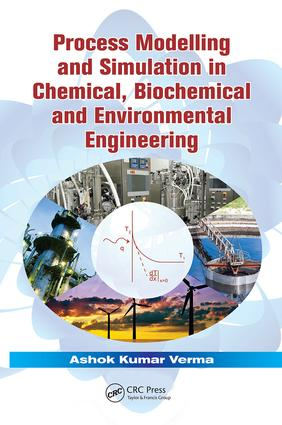Process Modelling and Simulation in Chemical, Biochemical and Environmental Engineering

1st Edition

CRC Press

424 pages | 85 B/W Illus.

Purchasing Options:\$ = USD
Paperback: 9781138075085
pub: 2017-03-30
SAVE ~\$12.99
\$64.95
\$51.96
x
Hardback: 9781482205923
pub: 2014-10-17
SAVE ~\$33.00
\$165.00
\$132.00
x
eBook (VitalSource) : 9780429090691
pub: 2014-10-17
from \$31.48

FREE Standard Shipping!

Description

The use of simulation plays a vital part in developing an integrated approach to process design. By helping save time and money before the actual trial of a concept, this practice can assist with troubleshooting, design, control, revamping, and more. Process Modelling and Simulation in Chemical, Biochemical and Environmental Engineering explores effective modeling and simulation approaches for solving equations. Using a systematic treatment of model development and simulation studies for chemical, biochemical, and environmental processes, this book explains the simplification of a complicated process at various levels with the help of a "model sketch."

It introduces several types of models, examines how they are developed, and provides examples from a wide range of applications. This includes the simple models based on simple laws such as Fick’s law, models that consist of generalized equations such as equations of motion, discrete-event models and stochastic models (which consider at least one variable as a discrete variable), and models based on population balance.

Divided into 11 chapters, this book:

• Presents a systematic approach of model development in view of the simulation need
• Includes modeling techniques to model hydrodynamics, mass and heat transfer, and reactors for single as well as multi-phase systems
• Provides stochastic and population balance models
• Covers the application and development of artificial neural network models and hybrid ANN models
• Highlights gradients based techniques as well as statistical techniques for model validation and sensitivity analysis
• Contains examples on development of analytical, stochastic, numerical, and ANN-based models and simulation studies using them
• Illustrates modeling concepts with a wide spectrum of classical as well as recent research papers

Process Modelling and Simulation in Chemical, Biochemical and Environmental Engineering includes recent trends in modeling and simulation, e.g. artificial neural network (ANN)-based models, and hybrid models. It contains a chapter on flowsheeting and batch processes using commercial/open source software for simulation.

Reviews

"Overall, the material covered is good. The need for mathematical modeling and simulation, the basic steps involved in the development of mathematical modeling and simulation, and validation of the models are highlighted a systematic way. For large number of situations, the associated mathematical model equations with the relevant boundary conditions/initial conditions are given. The analytical solutions, wherever possible, are given. For situations requiring numerical solutions, MATLAB® programs are given. There is a good presentation of the subject materials. The book can be recommended for two courses: one at the undergraduate level (chapters 1 to 4 and 9), and one at the post-graduate level (chapters 5 to 11)."

—Dr. M. Chidambaram, Indian Institute of Technology Madras

"A thorough book on modeling and simulation for different engineering fields that is augmented by case studies from a wide range of applications."

—Jadran Vrabec, Mechanical Engineering, University of Paderborn, Germany

"The strength of the book is the diversity of topics covered starting with models based on simple laws and conservation laws illustrated for multiphase systems without and with reaction. …The whole picture of process modeling and simulation ends with the last chapter on simulation of large plants."

—Alirio E. Rodrigues, The Faculdade de Engenharia da Universidade do Porto, Portugal

Introduction to Modelling and Simulation

Chemical Processes

What Is Simulation?

Modelling

Summary

References

An Overview of Modelling and Simulation

Strategy for Simulation

Approaches for Model Development

Types of Models

Types of Equations in a Model and Solution Strategy

Sources of Equations

Simplifying Concepts

Summary

References

Models Based on Simple Laws

Equation of State

Henry’s Law

Newton’s Law of Viscosity

Fourier’s Law of Heat Conduction

Fick’s First Law

Fick’s Second Law

Film Model

Two-Film Theory

Arrhenius’ Law

Examples

Summary

References

Models Based on Laws of Conservation

Laws of Conservation of Momentum, Mass and Energy

Laminar Flow

Boundary Layers: Momentum, Thermal and Diffusional

Turbulence Models

Surface Renewal Models at High Flux of Momentum,

Mass or Heat

Analogy between Momentum, Mass and Heat Transfer

Simple Models for Reactors and Bioreactors

Summary

References

Multiphase Systems without Reaction

Consideration of a Continuous-Phase Axial Solid Profile in a Slurry Bubble Column

Single Interface: The Wetted Wall Column

Stationary Dispersed-Phase Systems (Gas–Solid Systems)

Moving Dispersed Systems (Gas–Solid Systems):

Wall-to-bed Heat Transfer in a Fluidised Bed

Moving Dispersed Systems (Gas–Liquid Systems): Transfer Processes in Bubble Columns

Regions of Interest Adjacent to the Interface

More Than One Mechanism of Heat Transfer: Flat-Plate

Solar Collector

Introducing Other Effects in Laws of Conservation

Summary

References

Multiphase Systems with Reaction

Development of a Model for Multiphase Reactors: Common Assumptions and Methodology

Packed Bed Reactors

Trickle Bed Reactors

Slurry Reactors

Fluidised Bed Reactors

Summary

References

Population Balance Models and Discrete-Event Models

Stochastic Models

The Complex Nature of the Dispersed Phase

Population Balance Equation

Probability Distribution Functions

Population Balance Models: Simulation Methodology

Summary

References

Artificial Neural Network–Based Models

Artificial Neural Networks

Development of ANN-Based Models

Applications of ANNs in Chemical Engineering

Advantages of ANN-Based Models

Limitations of ANN-Based Models

Hybrid Neural Networks

Summary

References

Model Validation and Sensitivity Analysis

Model Validation: Objective

Model Validation Methodology

Sensitivity Analysis

Global Sensitivity Measures

Role of Sensitivity Analysis

Summary

References

Case Studies

Axial Distribution of Solids in Slurry Bubble Columns: Analytical Deterministic Models

Conversion for a Gas–Liquid Reaction in a Shallow Bed: A Numerical Model

Stochastic Model to Predict Wall-to-Bed Mass Transfer in Packed and Fluidised Beds

Artificial Neural Network Model: Heat Transfer in Bubble Columns

Summary

References

Simulation of Large Plants

Interconnecting Sub-Models

Simulation Study

Flowsheeting and Continuous Processes

Short-Cut Methods and Rigorous Methods

Dynamic Simulation

Batch Processes

Summary

References

Appendix A

Appendix B

Index